Generalized Least Squares
In this chapter we generalize the results of the previous chapter as the basis for introducing the pathological diseases of regression analysis. First, we abandon the assumption of a scalar diagonal variance of the error term, but find that with certain modifications a least squares estimator is still BLUE. Subsequently we consider heteroscedasticity and autocorrelation. In these subsequent sections we examine the consequence of the violation of the classical assumption, the detection of the problem, and a proposed remedy for the problem.

The Aitken Estimator
As before we posit Y = Xb + U with r(X) = k < n and X is uncorrelated with the error term. But now we generalize the assumptions about the error term a little bit.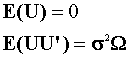We assume that the error term still has a mean of zero. Although the error variance is not scalar diagonal, we do know its structure up to a scalar constant. It remains symmetric and positive definite.
Under the present assumptions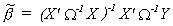is the BLUE for our posited model. We can prove this as follows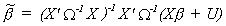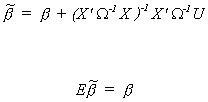So at the very least this estimator is unbiased and linear in Y.
Turning to the variance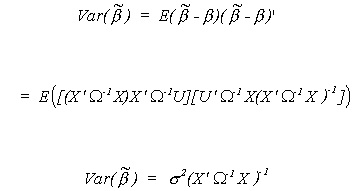Using the construction of the Gauss-Markov Theorem we could easily show that no other linear unbiased estimator has a smaller variance.
How about an estimator of the unknown s2? Well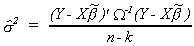is a natural since, by substitution,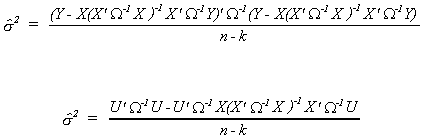shows our choice to be unbiased.
At the outset it was assumed that the error covariance matrix was known up to the scalar s2. This is a rather strong assumption. In its absence we have another n(n+1)/2 unknowns to estimate. This would in all likelihood be well beyond the capabilities of our data. The result is that we often make specific assumptions about the structure of the error covariance matrix. In any event, once we have made an assumption about the structure of W it may be possible to estimate it consistently. When we have a consistent estimator to use in place of W in the GLS estimator then we can show that the slope coefficient estimator is consistent.
The following curiosities are of more than passing interest.
(i) Because of the way regression packages are programmed they automatically and by default give you the following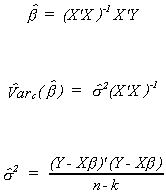Since this is the least squares estimator it remains unbiased, although the computer has given it to you inadvertantly. However, when the error covariance matrix is not scalar diagonal, the OLS estimator is no longer efficient.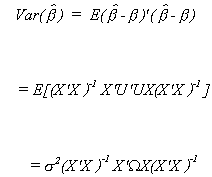The variance of the OLS estimator must be greater than that of the GLS estimator by the Gauss-Markov Theorem.
(ii) If plim(X'X)-1 = 0 then OLS is consistent.
(iii) We can also show that OLS is asymptotically normal.

Heteroscedasticity
While reading you should keep in mind several questions: What is heteroscedasticity? What are the consequences for OLS? How does one detect it? Ow does one correct for it? As a pathological disease, heteroscedasticity impacts the disturbance term. Typically we assume EUi2 = s2 for all i in the sample. That is, the error term is homoscedastic; all errors have the same variance.
Consider the case where we observe a number of firms at the same point in time. It is reasonable to expect that the error corresponding to larger firms will have a bigger variance than that for the smaller firms. Or consider attempts to estimate income elasticites on the basis of a cross section. For any commodity, we would expect more variability in consumption from the higher income group.
We shall begin with a rather general discussion. We posit

Y = Xb + U
and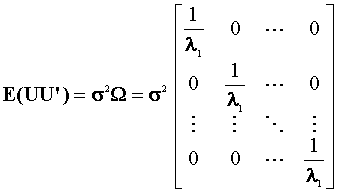we will assume s2 is not known and W is a known, symmetric and positive definite matrix. Now define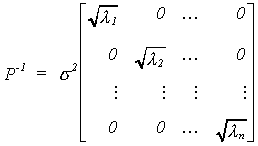Then P-1'P-1 = W-1.
According to our previous work with the Aitken estimator we find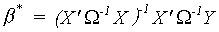to be BLUE and its variance is given by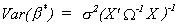If we already know W then we should transform the model by P-1. That is P-1Y = P-1Xb + P-1U
So that when we construct the least squares estimator from the transformed data we getIt is only in special circumstances that we know the specific form of W.
When the error covariance is not scalar diagonal and we apply OLS anyway the estimator is not efficient. That is, there is a linear unbiased estimator with smaller variance. The inadvertant use of OLS, and its attendant large standard errors (small t statistics) will lead us to conclude that a variable is not significantly different from zero.
An example of correcting for heteroscedasticity follows.

EXAMPLE
Consider a standardized exam and parent's income. We propose

qij = a + bxij + uij (1)

for i = 1, 2, ... , nj and j = 1, 2, ... , m
where qij is the test score of a particular student from the jth school and xij is his parent's income. There are m schools with nj students in each. The error term is thought to be homoscedastic.

As a result of privacy laws we do not observe individual scores and income. Rather, we observe the average score for the school.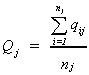is the average score of the students in the school and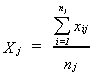is the average income of parents in school j.
Note that the schools are of different sizes. Therefore it will be necessary to weight the observations.
The model we are really using is

Qj = a + bXj + Uj (2)

j = 1, 2, ..., m

The error term in the more crass model is characterized by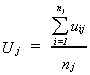Because the denominator is different for each school, the variance of each Uj will differ. While OLS will still be unbiased, it will not be efficient. What can we do to make our estimates of a and b as good as possible? Begin by finding the error variance for the crass model.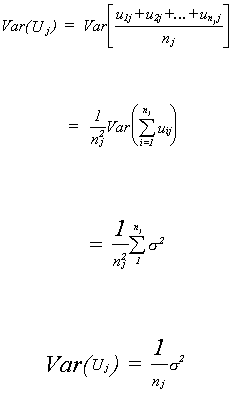Using these results to put together the whole error covariance matrix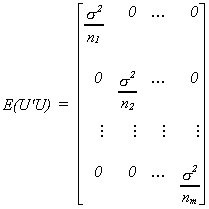We must correct for the different variances on the diagonal. If we properly weight the observations we can get a scalar diagonal covariance matrix. Define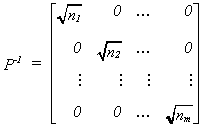Now transform the data as follows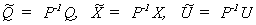By rescaling the data in this fashion we arrive at the following conclusion regarding the error term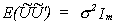Therefore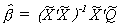is BLUE.

Testing for Heteroscedasticity

Goldfeld Quandt Test
It behooves us to have a method for determining when we have the disease of heteroscedasticity. Goldfeld and Quandt suggest the following test procedure.
1. Choose a column of X according to which the error variance might be ordered.
2. Arrange the observations in the model in accordance with the size of Xj.
3. Omit an arbitrary number, say c, of central observations from the ordered set. Make sure (n-c)/2 > k.
4. Fit separate regressions to the first (n-c)/2 observations and to the next (n-c)/2 observations.
5. Define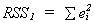for the residuals from the case where Xj are small and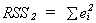for the residuals from the case where Xj are large.
6. Let F = RSS2/RSS1. This has an F distribution with (n-c)/2-k degrees of freedomn on numerator and denominator.
The hypothesis we are testing is
Ho: F = 1
H1: F > 1
If we observe a large F then we reject the null.

Replicated Data
This particular problem is most often seen in the natural sciences. A classic example to be found in economics is Nerlove. The idea is that we have several observations (i=1,2,...,ni) for each size class of firms and there are m size classes. The model is yij = a + bxij + uij i=1,2,...,m; j=1,2,...,ni with the assumptions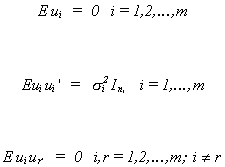If we stack the data then the model is written as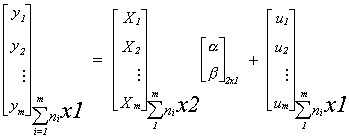The subscripts on the braces show the dimensions of the various matrices. Note the implied restriction that the intercept and slope are equal across groups. Look for this question to come up in the sections on seemingly unrelated regression and random effects models. In this revised form the error covariance for the entire disturbance vector is written as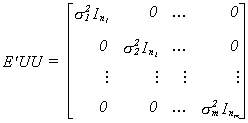The hypothesis to be tested is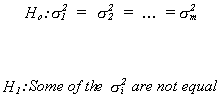The test procedure is as follows
1. Pool the data and estimate the slope parameters by OLS.
2. From the entire residual vector, e, estimate the pooled least squares residual variance,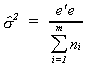Note that you are using the maximum likelihood estimate of the error variance under the null hypothesis.
3. For each group or class in the sample construct an estimate of the error variance from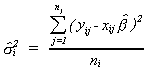4. Construct the test statistic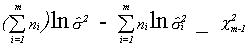5. For large observed test statistics we reject the null hypothesis.

Glejser Explorations

Glejser offers an approach to the heteroscedasticity problem based on running some supplementary regressions.
Procedure:
1. Estmate the parameters of Y = Xb + U. Obtain the least squares residuals, ei.
2. Estimate the parameters of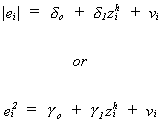3. Construct a test of the hypothesis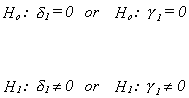Breusch-Pagan
Breusch and Pagan offer an extension to the work of Glejser. Essentially they provide a test to help the researcher decide whether or not s/he has solved the heteroscedasticity problem.
Once again the model is Y = Xb + U, but with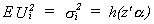the a are p unknown coefficients and the zi are a set of variables thought to affect heteroscedasticity. The null hypothesis is that apart from the intercept the ai are all zero, Ho: a2=a3= ... =ap=0.

Procedure:
1. Fit Y = Xb + U and obtain the vector of OLS residuals, e.
2. Compute the maximum likelihood estimator for s2 and a variable that we will use as dependent variable in a supplementary regression.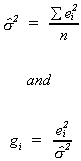3. Choose the variables zi then estimate the coefficients of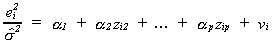and obtain the residuals.
4. Compute the explained sum of squares from the regression in step 3.
5. From the explained sum of squares construct the test statistic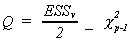The null hypothesis of homoscedasticity is rejected for large values of Q.

White's General Test
White's test has become ubiquitous. It is now programmed into most regression packages, both the test and the correction. The correction computes the proper estimate of the variance when one applies OLS in the presence of heteroscedasticity. This correct variance is then used in tests of hypothesis about the slope parameters.
Recall that the correct covariance matrix for the least squares estimator is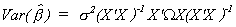. This can be consistently estimated by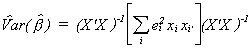where xi is the transpose of the ith row of X, so it has dimension kx1 and xi' has dimension 1xk. The default estimator used by most regression packages is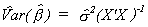. Which is, of course, not consistent when the errors are heteroscedastic.
To conduct the test for homoscedasticity use the following procedure
1. Apply OLS to the original model and construct ei2 from the residuals.
2. Estimate the parameters of the following regression model: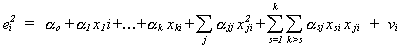The set of right hand side variables are formed by finding the set of all unique variables formed when the original independent variables are multiplied by themselves and one another.

The test statistic is formed from the simple coefficient of determination in step 2. That is,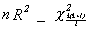.

Spearman's rank Test

A nonparametric test is offered by Spearman. Suppose you have the model yi = xib+ui. First estimate the model parameters and save the residuals. For the sake of the example, we believe the variance of ui to be related to the size of the observation on x. If they are directly related then the rank of the ith obervation on x, say dix, should correspond to the observed rank diu. Hence, one would expect the differences in the ranks for x and u to be zero on average. Let the squared difference for the ith observation be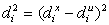and construct the correlation coefficient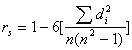.
If the rank orderings are identical for x and u, then rs will be one.
As with any correlation coefficient, we can do a t-test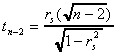Autocorrelation

As a pathological disease, autocorrelation also impacts the disturbances. Contrary to the original assumption about our regression model, we now allow the error in one period to affect the error in a subsequent period. Consider the model Yt = Xtb + Ut where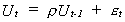is the serially correlated error. We assume the following about et and r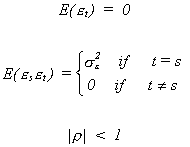The et term is known as white noise; it has constant mean and variance for all periods and is not serially correlated. Since r is nonzero it introduces some persistence into the system when there are shocks from the white noise term.
We can expand our expression for Ut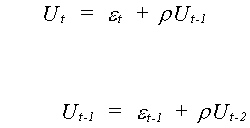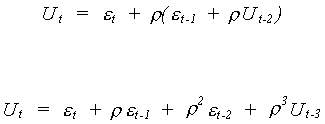By continuous substitution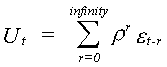The current disturbance is a declining average of the entire history of the white noise term. In order to say anything about the use of OLS in the presence of autocorrelation we need to find the mean and variance of the disturbance.
MEAN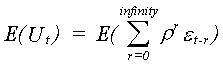Since the RHS is a linear combination of random variables all with mean zero, the disturbance has a mean of zero.
VARIANCE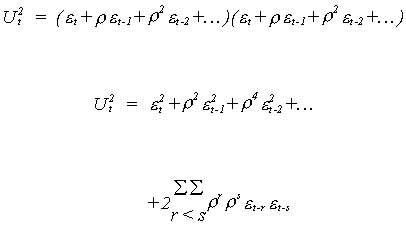Upon taking expectations the terms under the double sum are all cross products and drop out. That is, their time subscripts do not match and we have assumed that there is no serial correlation in the white noise term. Thus, using a bit of our human capital about infinite series,We also need to be able to say something about the covariance between the current disturbance and past disturbances in order to build up the entire error covariance matrix for the sample.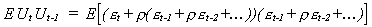Note the following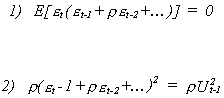Applying the same tricks of the trade that we used for the expectation of Ut2 we find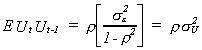We could apply the same tricks ad nauseum for all the possible offsets in time subscript and arrive at the following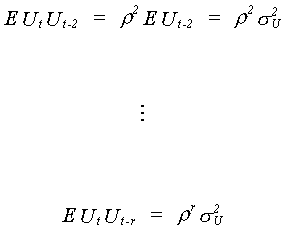Putting everything together in an error covariance matrix gives us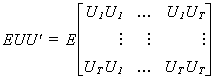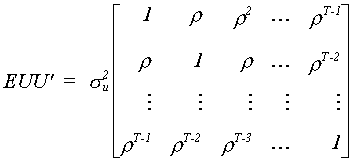Let us now consider the effects of autocorrelation on our conventional OLS estimator.

Consequences of Autocorrelation for OLS
BIAS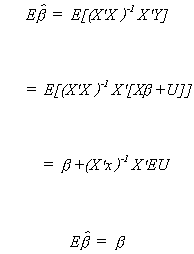Therefore autocorrelation leaves OLS unbiased.

VARIANCE OF OLS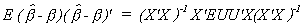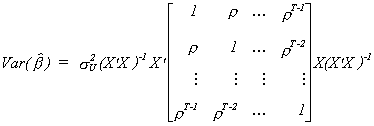In order to shed more light on this we will consider the very simple model yt = bxt + ut with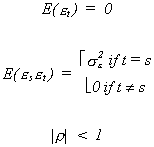and make x a column vector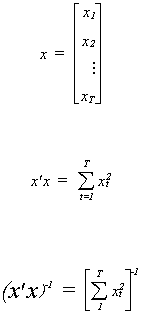Using our earlier results on the form of the covariance matrix for the OLS estimator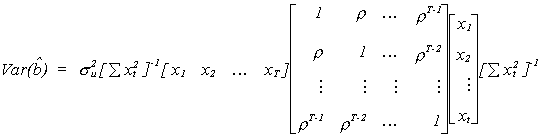If you persist and do the multiplication then once the smoke clears you will arrive at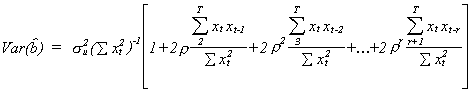Recall that your regression package always reports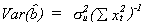for the variance of the least squares estimator. Which is larger, the variance reported by the machine or the correct variance of the OLS estimator?
In the usual autocorrelation case 0 < r < 1. If you look closely at each term of the variance for our example you will see that each fraction looks like the estimate of a regression coefficient. That is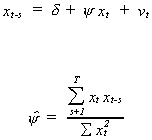Usually 0 < y. In fact, it is often the case with economic time series that we find y is approximately one. We conclude then that the machine reported estimate of the variance understates the true variance. The implication is that if we fail to detect and correct for autocorrelation and rely on the machine reported coefficient covariance then
o when calculating confidence intervals they will appear more precise than they really are
o we will reject more Ho than we should.
In spite of any problems with the dunderheaded computer, OLS remains unbiased. If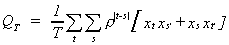, where xt' and xs' (of dimension 1xk) are rows of X, converges to a matrix of finite elements then OLS is consistent. The import of this requirement is that you had better not include a time trend in your time series model! The OLS estimator also remains asymptotically normal under most circumstances. The caveat emptor, as in the heteroscedasticity case, is that OLS is not efficient.

Testing for Serial Correlation

Durbin-Watson Test for Autocorrelation
D-W suggest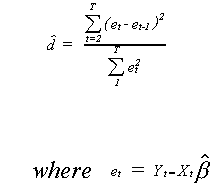D-W have shown the following
1. When r = 0 then = 2.0
2. When r > 0 then < 2.0
i.e. positive autocorrelation
3. When r < 0 then > 2.0
i.e. negative autocorrelation
4. 0 < < 4
Unfortunately, the distribution of depends on the matrix of independent variables, so is different for every data set. We can characterize two polar cases.
A. X evolves smoothly. That is, the independent variables are dominated by trend and long cycle components. If you regress a given variable on its own one period lag the slope coefficient would be positive.
B. X evolves frenetically. That is, the independent variables are dominated by short cycles and random components. If you regress a given variable on its own one period lag the slope coefficient would be negative.
For the case when r > 0 the situation is pictured below.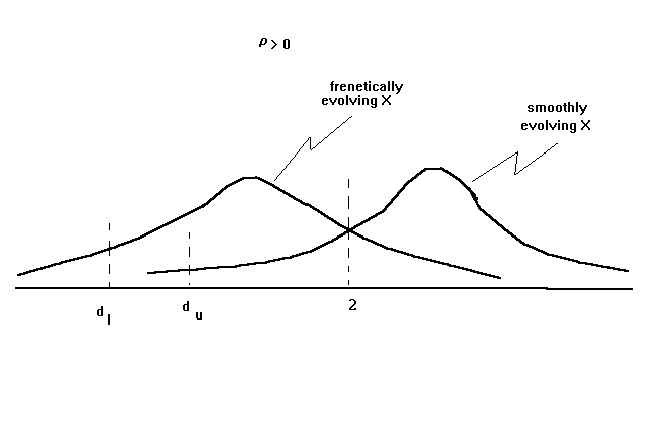As a result of the two polar cases there will be two critical values for the test statistic, dl and du. Since we never know whether our data evolves smoothly or frenetically we have a "no man's zone" in the reject region. If the observed value of the Durbin Watson statistic is less than dl, then we can state unequivocally that the null should be
rejected. If the observed Durbin-Watson is above du then we can state unequivocally that the null should not be rejected. But if is between dl and du then we must punt.
Apart from the "no man's zone", there are a few other problems with the Durbin Watson statistic. First, the null is against a specific alternative. The consequence is that if the error process is something other than AR(1) then the DW is easily fooled. Secondly, the DW critical tables are set up assuming that the researcher has included an intercept in his model. Thirdly, one cannot use the DW to test for an AR(1) error process if the model has a lagged dependent variable on the right hand side.
When there is a Lagged Dependent Variable
Suppose we have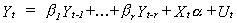with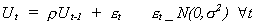Then the appropriate test statistic is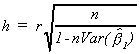where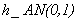and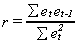.

Wallis' Test for Fourth Order Correlation
Suppose we have a quarterly model, then the error specification is likely to be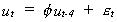and we wish to test the null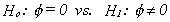. The test statistic will be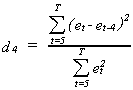The tabulated critical vaoues are in Wallis, Econometrica, Vol. 40, 1972 or Giles and King, Journal of Econometrics, Vol. 8, 1978.

Breusch-Godfrey Tests Against More General Alternatives

The null hypothesis is that the model looks like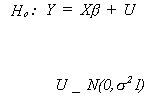The alternatives against which we can test are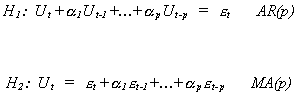where, under the alternatives,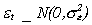.

Procedure
1. Construct the OLS residuals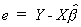.
2. Construct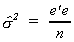the MLE for su2 and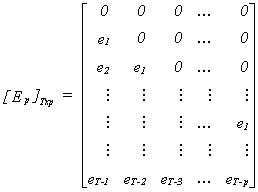3. Construct the test statisticreject the null for large values of the test statistic.
NOTE: The part in square brackets is a pxp matrix. The elements on the main diagonal are residual sums of squares from the regression of the columns of Ep on the column space of X. With this in mind, the procedure outlined here is equivalent to checking TR2 for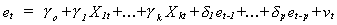against an appropriate critical value in the c2 table.

What to Do About Autocorrelation {AR(1)}

Although we will do the case in which the error term is AR(1), it is true that AR(1) and MA(1) are locally equivalent. The basic model is Yt = Xtb + Ut with Ut = Ut-1+t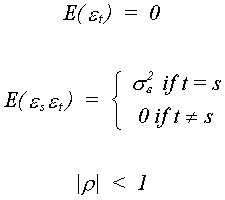Begin with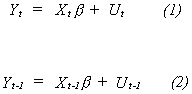Substitute the error structure into (1) and multiply (2) by r and subtract the result from (1) to get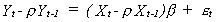Since Eet = 0 and Eee' = s2I we have an equation with no autocorrelation. If we knew r we could easily estimate the parameters of this well behaved model.
Define DYt = Yt - Yt-1, the first difference, and DrYt = Yt - rYt-1, the partial difference.

DURBIN'S METHOD

The well behaved model is Yt - rYt-1 = (Xt - rXt-1)b + et,
rewrite this as Yt = rYt-1 + Xtb - rXt-1b + et
Estimating the parameters of this model gives an estimate of r. Use the estimate of r to construct the partial differences and reestimate the model parameters. The Durbin process is best for small samples.

TWO STEP COCHRAN-ORCUTT METHOD

1. Estimate the model parameters with OLS.
2. Calculate an estimate of r from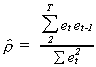3. Partial difference all of the data using the estimate of r.
4. Estimate the model parameters of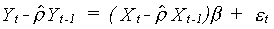using OLS.